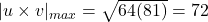## Let v = 8j and let u be a vector with length 9 that starts at the origin and rotates in the xy-plane. Find the maximum and minimum values of

Question

Let v = 8j and let u be a vector with length 9 that starts at the origin and rotates in the xy-plane. Find the maximum and minimum values of the length of the vector u × v.

in progress 0
1 month 2021-08-15T21:19:34+00:00 1 Answers 0 views 0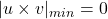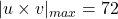Step-by-step explanation:

We are given that

v=8 j

|u|=9

Let u=ai+bj

We have to find the maximum and minimum values of the length of the vector

u × v.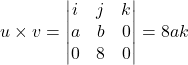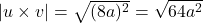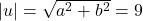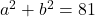The minimum value of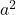=0

Then,Maximum value of=81